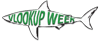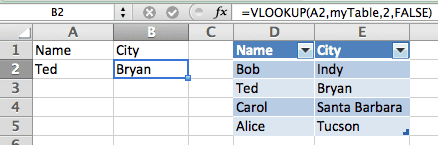# The VLOOKUP Function – Inside OutAs part of Shark Week I’ve committed to write something for VLOOKUP week. (It’s what I get for using twitter.) So without further ado.

I love the VLOOKUP Function in Excel. As the name implies, it’s a vertical lookup. Meaning the function will lookup data in columns.

## The VLOOKUP Function Arguments

The VLOOKUP function has four arguments and in my opinion the fourth argument always gets overlooked, yet it’s the first thing you need to know. So, like reverse polish notation, we’ll start from the inside and work out to explain each argument.

VLOOKUP(lookup_value,table_array,col_index_num,range_lookup)

### range_lookup

The range lookup argument it either TRUE or FALSE. I use FALSE 98% of the time, because using FALSE means the VLOOKUP function will find an exact match. If no match is found then it returns the #N/A error value. Note: And by exact, they mean EXACT. An extra space character, which is not visible to the naked eye, will cause and error.

The strange thing is that you don’t even need the fourth argument, but if it’s missing the default value is TRUE. Bad choice by my estimation, but that because I rarely use TRUE. If the range lookup value is TRUE then the VLOOKP function will produce an approximate match.

This can be quite handy if you want to return something like grades, you know: A, B, C, D, F, when given a numerical value between 0 and 100. Teachers must love TRUE. The only catch here is that the first column of the lookup table has to be sorted in ascending order. You can find out more here.

### col_index_num

This argument is just the column number from the table you are looking up. However, this column will contain the data you want the VLOOKUP function to return. For example, I have a table with Names in the first column and Cites in the second column. I want the VLOOKUP function to return the City value so the index number is 2, for the second column in the table.

### table_array

The table is where VLOOKUP gets its information. This is where the data is looked up. The reference to the table may take several forms. You normally use an absolute range reference, like \$A\$1:\$B\$5. In Excel 2003 I like to use a defined Range Name. In newer versions of Excel I use a TABLE to store the information, and hence the Table Name is what I use for the second argument.

### lookup_value

We finally come to the lookup_value. This is a single reference the VLOOKUP function uses to find a match in the first row of the Table. For example, if I want to lookup a Name and find the corresponding City, the lookup_value should reference a name and the first column of the table should be a column of names.

### VLOOKUP Function in Action

In the screen shot below you can see the VLOOKUP function shown in the formula bar, which is for cell B2.

=VLOOKUP(A2,myTable,2,FALSE)

I’m using FALSE in the fourth argument for an exact match. For the third argument, I want to return data from column 2 of the Table, which is for the City. For the second argument, the reference is the Table name myTable, which is the range D1:E5. Finally, the the first argument, A2 is a reference to a name.So the VLOOKUP function in cell B2, looks in cell A2 and finds a name (Ted), then goes to the table, myTable, and locates an exact match in the first column (row 3), then goes over to the second column and returns that value (Bryan) to cell B2.

Note: The myTable reference refers to the range D2:E5, and doesn’t include the header row.